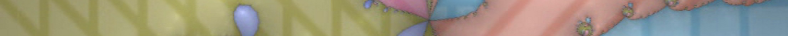# mathr

##newton

### Newton's method for periodic cycles

Reject lower periods, and iteratively perturb a known cycle along a path.

### Newton's method for periodic points

Rejecting lower periods by division, instead of checking later.

### Calendar 2015 - Two Beetles Meet Where Land Meets Sky

Distance estimated Newton fractal for a rational function with zeros at {1, i, -1, -i} and poles at {-2, 2}.

### Newton's method for Misiurewicz points

Rejecting lower preperiods instead of checking later.

### Practical interior distance rendering

Using Newton's method.

### Perturbation glitches

Rebasing from a reference point.

### Mandelbrot Notebook

Handwritten notes on various properties.

### Ultimate Anti-Buddhabrot

An xmas present for someone special.

### Distance estimation for Newton fractals

Derived from distance estimation for Julia sets.

### Preperiodic Mandelbrot set Newton basins

finding tuned branch points

### Mandelbrot set Newton basins

speeding up external ray tracing

### Fractal Juggling